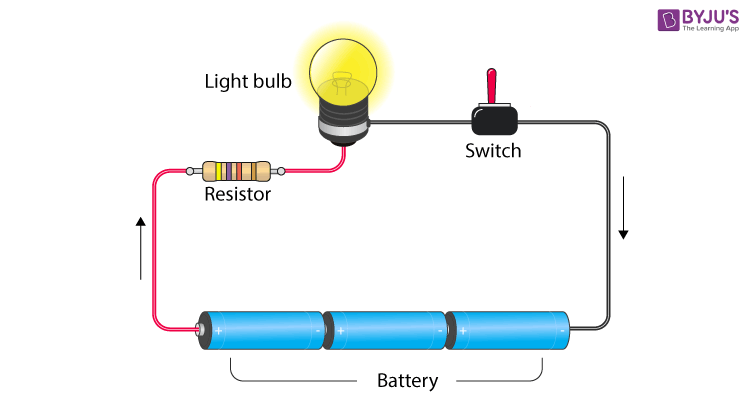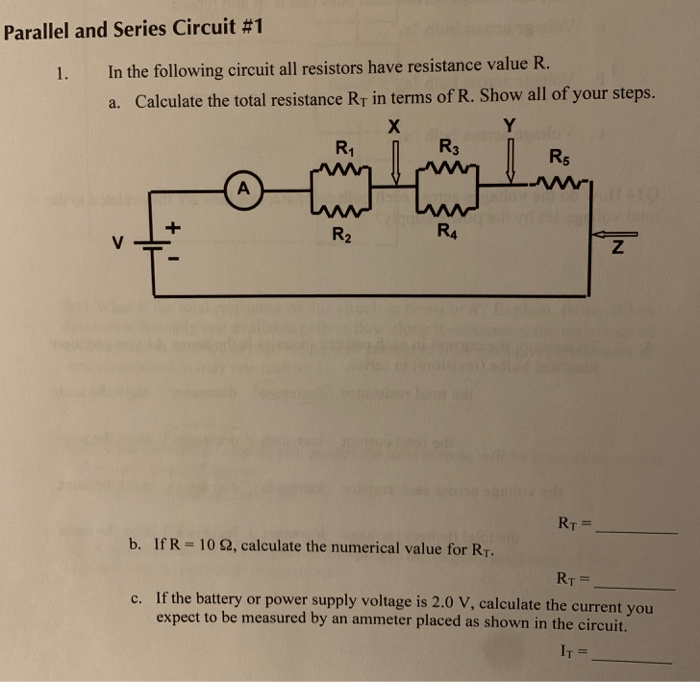# How To Calculate Resistance Value In Series And Parallel Circuits

By | September 22, 2023

Electricity is the backbone of our modern world, powering everything from our homes to our technology. But have you ever wondered how it all works? The answer lies in circuits – specifically, series and parallel circuits. Understanding these two types of circuits is crucial for anyone who wants to tinker with electronics or simply have a better understanding of how electricity works. And one important aspect of circuits is calculating resistance value. In this article, we will delve into the world of series and parallel circuits and learn how to calculate resistance value in each type.

First, let's define what series and parallel circuits are. In a series circuit, all components are connected one after another, creating a single path for electricity to flow through. This means that the current remains the same throughout the circuit, but the voltage drops as it passes through each component. On the other hand, in a parallel circuit, the components are connected side by side, creating multiple paths for electricity to flow through. Here, the voltage remains the same, but the current is divided between the components.

So, why is calculating resistance value important in these circuits? Resistance is the measure of how difficult it is for electricity to flow through a component. In series circuits, the total resistance is equal to the sum of individual resistances, while in parallel circuits, it is calculated using a different formula. Knowing the resistance value is crucial in determining the overall performance of the circuit and ensuring that the components do not get damaged due to excessive current.

To calculate resistance in a series circuit, you simply add up the values of each component. For example, if you have three resistors with values of 10 ohms, 20 ohms, and 30 ohms, the total resistance would be 60 ohms. On the other hand, calculating resistance in a parallel circuit is a bit more complex. Here, you use the inverse of the sum of the inverse values of each component. This means that the total resistance will always be lower than the lowest individual resistance value.

In conclusion, understanding series and parallel circuits and how to calculate resistance value is crucial for anyone interested in electronics. Whether you're a hobbyist or a professional, having a strong grasp of this fundamental concept will open up a world of possibilities for you. So, the next time you're tinkering with your electronic gadgets or trying to fix a faulty circuit in your home, remember the importance of resistance value and how to calculate it accurately.Series Parallel Resistors Formulas Calculator Electronics NotesSeries And Parallel Ap Physics 1Physics Tutorial Parallel CircuitsPhysics Tutorial Series CircuitsResistors In Series And Parallel Circuit Components S FaqsHow To Calculate The Voltage Drop Across A Resistor In Parallel CircuitSolved Parallel And Series Circuit 1 In The Following Chegg ComSeries And Parallel CircuitsSimplified Formulas For Parallel Circuit Resistance Calculations Inst ToolsSimplified Formulas For Parallel Circuit Resistance Calculations Inst ToolsElectrical Electronic Series CircuitsResistors In Parallel Resistor Applications GuidePhysics Tutorial Combination CircuitsSimple Series Circuits And Parallel Electronics TextbookSeries And Parallel Circuits Learn Sparkfun ComCalculate The Value Of Resistance R2 In Parallel Circuit BrainlySimple Parallel Circuits Series And Electronics TextbookSolved Measurement Of Resistances In Series And Parallel Connect The Circuit Shown Figures Ri R2 Are R3 R4 1ov Fig 5 Diagram

4.5